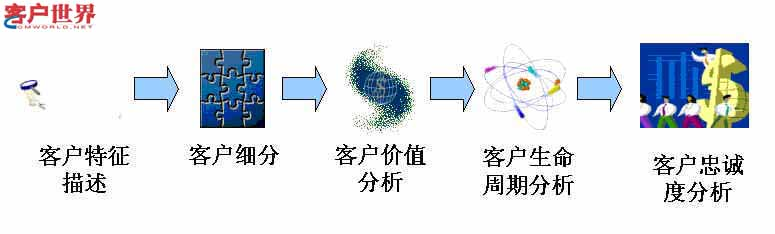# CRM已死，CRM重生

|     2015年7月12日   |   2005年   |     评论已关闭   |    1137

200110月深圳招商地产开始CRM尝试至今，房地产行业的CRM实践已经走过三个年头。细数起来，已经有十几家房地产企业部署了CRM系统，分布在全国各地，如深圳、广州、上海、北京、杭州、大连、成都、济南等，最密集的当然属房地产市场最成熟的深圳地区。一时间，很多人都为房地产行业的CRM实践欢喜鼓舞，一致认为房地产CRM应用进入到令人欣喜的阶段，大有高歌猛进的势头。

CRM泡沫的原因

CRM泡沫的原因

CRM真知

CRM完整过程

CRM强调的是一个对客户完整了解的过程，在这个过程中，贯穿着以客户为中心的主线，而这也是企业运营流程所应该围绕的中心。整个过程以客户细分为基础，基于客户细分的不同通过差异化的影响渠道提供差异化产品和服务。当企业发展到一定规模，业务开始跨产品、跨区域、跨目标客户群进行操作的时候客户细分就越显得重要。如果一个企业的CRM项目没有进行客户细分，而仅仅是部署CRM系统，就如同空中楼阁水中花月。我们先来看一下CRM的完整过程：< p align="center">
< p align="left">客户细分与客户价值
< p align="left">
< p align="left">
< p align="left">
< p align="left">国内企业的发展正在经历三个阶段：产品导向、市场导向和客户导向。大部分国内企业还处在产品开发为主的产品导向阶段和市场细分定位的市场导向阶段，还没有真正进入以基于客户细分的客户价值和客户需求导向的阶段。
< p align="left">
< p align="left">
< p align="left">
< p align="left">在没有导入客户细分战略的时候，国内企业的运营流程面对的是全部客户，或者是假想的市场群体，没有做到一对一营销，而是面向泛泛客户的营销、销售和服务。以产品为中心的传统思维模式，考虑产品卖给谁、怎么卖出高价格获取高额利润，无法长期有效维系客户忠诚度和挖掘客户价值，企业短期但表面的胜利无法长久下去。
< p align="left">
< p align="left">
< p align="left">
< p align="left">导入客户细分战略，可以有效的基于客户细分的基础来贯串整个企业的运营流程，如设计什么样的产品、策划什么样的营销方案、进行什么样的销售管理、提供什么样的服务等，都可以基于客户细分后的客户需求来体现。客户细分有三大亮点：基于价值进行科学的客户细分、基于战略确立广泛的客户定位、将客户细分与精细化的运营流程紧密连接。
< p align="left">
< p align="left">
< p align="left">
< p align="left">客户消费行为
< p align="left">
< p align="left">
< p align="left">
< p align="left">了解客户，不仅仅是了解客户的信息和需求，更重要的是建立起对客户的架构性认识，即从归纳的角度来模拟和实现客户的消费曲线和消费模型，从而准确的预测客户的消费趋势和消费模型，当然这些分析都是基于一个完善的客户细分的基础上的。对所有受众的消费模型分析是没有意义的，而对一个细分的客户区隔的消费习惯的分析和再现是有指导意义的。
< p align="left">
< p align="left">
< p align="left">
< p align="left">客户的消费曲线模型是从客户的点点滴滴消费信息积累分析而来，包括客户的特征、心理、意向、状态变化等信息，在不同营销阶段和销售阶段所体现出来的消费反应，可以经过归纳提炼映射在消费曲线模型上。
< p align="left">
< p align="left">
< p align="left">
< p align="left">在客户的完整生命周期内，一个客户创造的价值不仅仅是现在的客户价值，还包括未来可能产生的潜在价值，以及在市场营销活动的促进下产生的增量客户价值。因此，对客户在一个完整的消费生命周期内的消费习惯和特征、趋势进行分析归纳，可以有效的指导差异化营销和服务。
< p align="left">
< p align="left">
< p align="left">
< p align="left">客户导向的销售能力提升
< p align="left">
< p align="left">
< p align="left">
< p align="left">国内企业大部分没有真正重视客户细分和客户开发，CRM系统提供多角度的客户细分，并根据企业关注的多种要素对客户进行分级，不同级别的客户在销售团队的客户拜访计划中就有针对性的体现，包括时间段、时间长、资料准备等方面。
< p align="left">
< p align="left">
< p align="left">
< p align="left">现在的营销越来越注重主动出击销售。一个客户拜访计划并非简单的事情，而是有一个严格的步骤：客户分析－客户分类－确定客户访问次数和频率－设计访问线路－设计工作进度表－访问客户－填写销售日报表（操作CRM系统）－客户拜访评估。通过这样科学的步骤，才能实现有效的客户拜访，从而实现改善销售人员时间利用率，提高每次访问工作效率，进而有利于大客户的开发，有助于减少销售费用，最终提高销售人员的业绩。
< p align="left">
< p align="left">
< p align="left">
< p align="left">在复杂的项目销售中，企业可以根据客户细分，设置不同的销售小组来满足不同细分客户区隔的特别需求；而在客户细分的基础上，识别出最有价值的客户群，使精干的销售队伍和销售资源能够配合这些有决定权和影响力的客户群；同时通过激励、合理的工作安排，提高销售小组的团队精神，从而提高销售队伍力量，满足客户日益复杂的要求。
< p align="left">
< p align="left">
< p align="left">
< p align="left">真正强大灵活的CRM支持工具
< p align="left">
< p align="left">
< p align="left">
< p align="left">灵活性是国内企业CRM系统部署的关键因素，CRM使用者尤其是销售代表大多工作时间不在电脑旁边，因此对移动终端如手机、PDA等的支持显得极为重要，而不是现在CRM厂商鼓吹的浏览器结构就足矣。
< p align="left">
< p align="left">
< p align="left">
< p align="left">扩展性是国内企业实现个性化特点定制的保障，企业在发展中不断产生的新的个性化特点如何及时有效的反馈进CRM系统，而不是依赖于CRM厂商的二次研发，这就要求CRM系统是基于一个可以充分扩展和灵活定制的平台。
< p align="left">
< p align="left">
< p align="left">
< p align="left">可操作性是CRM使用者的期望，而这个期望实现并非难事，大部分使用者都习惯了微软应用软件如Word、Excel的操作，那么类似微软应用软件的友好操作性是提高CRM使用者的操作情绪的关键因素（有时候奖罚并非最佳选择）。
< p align="left">
< p align="left">
< p align="left">
< p align="left">集成性是企业CRM的基础，客户细分和客户消费行为分析需要有机的集成售楼、租赁、物业、客服、会员等业务系统，只有当CRM有机的集成并融合了业务系统之后，对客户的整体分析和管理才成为可能。但为了集成业务系统而丢弃CRM核心和战略架构也是不可取的，这种畸形现象在目前的实践中非常突出。
< p align="left">
< p align="left">
< p align="left">强大的分析功能，对于企业战略层面的管理需求，不是希望看到那些静态的报表，而是基于客户战略和流程的分析模型，比如客户细分矩阵模型、客户价值矩阵模型、营销投资回报模型、客户满意度与忠诚度模型等。
< p align="left">
< p align="left">
< p align="left">
< p align="left">CRM重生
< p align="left">
< p align="left">
< p align="left">
< p align="left">企业需要的不是CRM软件解决方案，而是真正的以客户为中心，对有价值的客户给予更多的关注。然而，很多已经部署了CRM系统的企业的现实与期望总是存在差距，而且可能是巨大的差距。要真正让CRM重生，就要从根本上来优化企业的客户关系管理解决方案。
< p align="left">
< p align="left">
< p align="left">
< p align="left">企业现有的客户关系管理解决方案的优化步骤可以简单分为四个阶段：
< p align="left">
< p align="left">
< p align="left">
< p align="left">确定企业业务经营的关键因素，进行企业业务经营的市场分析、竞争分析、基于客户特征的客户细分等；
< p align="left">
< p align="left">评估客户价值，进行基于客户价值的定性和定量评估；
< p align="left">
< p align="left">
< p align="left">
< p align="left">设计、测试和应用客户管理价值策略，进行CRM分析、客户消费行为和需求、数据智分析、ROI以及客
< p align="left">满意度与忠诚度等客户管理价值策略；
< p align="left">
< p align="left">
< p align="left">
< p align="left">测评、分析和优化客户关系管理流程，检验CRM系统、建立价值评估模型、测评成效、优化解决方案。
< p align="left">
< p align="left">
< p align="left">
< p align="left">我们欣喜的看到，国内的企业和系统集成商们都在不断探索和实践，而这凤凰重生的美好一天必将来到！
< p align="left">
< p align="left">
< p align="left">
< p align="left">本文刊载于《客户世界》杂志2005年4月刊；作者为大中华客户关系管理研究所所长
< p align="left">